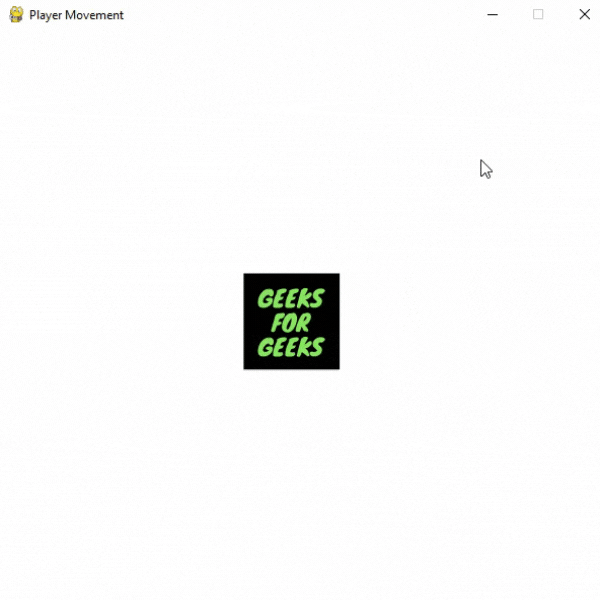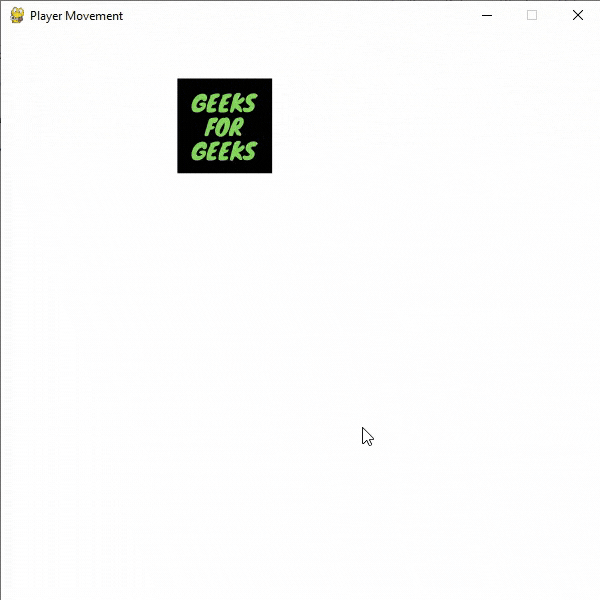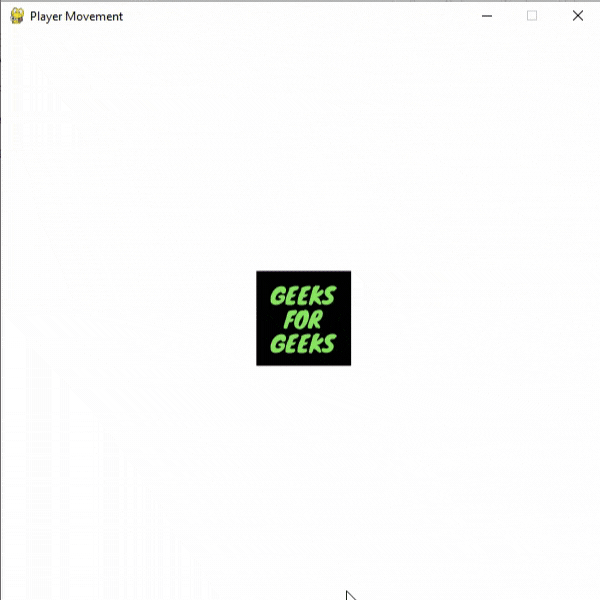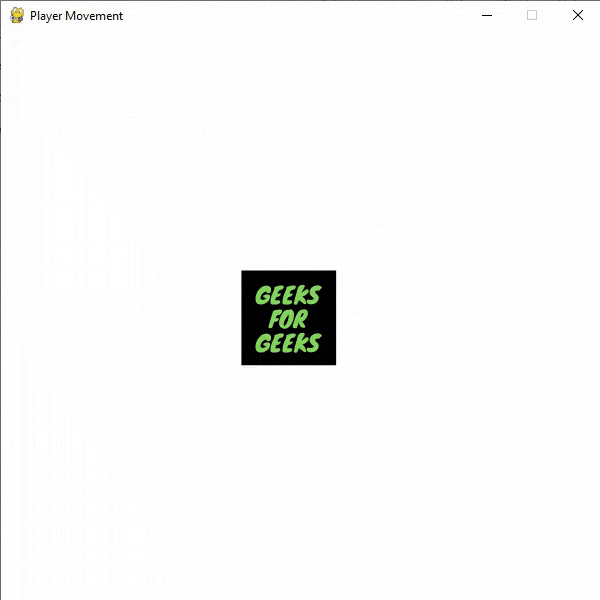Related Articles

# How to move your Game Character Around in Pygame

• Difficulty Level : Hard
• Last Updated : 23 Aug, 2021

Prerequisites: Pygame

Pygame is a cross-platform set of Python modules designed for writing video games. It includes computer graphics and sound libraries designed to be used with the Python programming language. You can create different types of games using pygame including arcade games, platformer games, and many more.

Images in use:You can control your player’s movement. For this first create a display surface object using display.set_mode() method of pygame and add player’s sprite using image.load() method of pygame. The set_mode() function is used to initialize a display surface or window. The size argument is a pair of numbers representing the width and height. The flags argument is a collection of additional options. The depth argument represents the number of bits to use for color.

Syntax:

set_mode(size=(0, 0), flags=0, depth=0, display=0, vsync=0)

Create a variable to store the velocity of the player. Set the initial coordinates of the player. Now, change the x and y coordinates of the player according to the keyboard events i.e. events that occur when the state of key changes.

blit(surface, surfacerect) function is used to draw the images on the screen.

Syntax:

blit(surface, surfacerect)

For collecting all the events from the queue get() function of the event module is used then we are iterating over all the events using a for loop.

Syntax:

get(eventtype=None)

Updating the screen using the update() function of the display module.

Syntax:

update(rectangle=None)

Below is the implementation.

Example: Program for player movement

## Python3

 `# Importing pygame module``import` `pygame``from` `pygame.``locals` `import` `*`` ` `# initiate pygame and give permission``# to use pygame's functionality.``pygame.init()`` ` `# create the display surface object``# of specific dimension.``window ``=` `pygame.display.set_mode((``600``, ``600``))`` ` `# Add caption in the window``pygame.display.set_caption(``'Player Movement'``)`` ` `# Add player sprite``image ``=` `pygame.image.load(r``'Player_image.png'``)`` ` ` ` `# Store the initial``# coordinates of the player in``# two variables i.e. x and y.``x ``=` `100``y ``=` `100`` ` `# Create a variable to store the``# velocity of player's movement``velocity ``=` `12`` ` `# Creating an Infinite loop``run ``=` `True``while` `run:`` ` `    ``# Filling the background with``    ``# white color``    ``window.fill((``255``, ``255``, ``255``))`` ` `    ``# Display the player sprite at x``    ``# and y coordinates``    ``window.blit(image, (x, y))`` ` `    ``# iterate over the list of Event objects``    ``# that was returned by pygame.event.get()``    ``# method.``    ``for` `event ``in` `pygame.event.get():`` ` `        ``# Closing the window and program if the``        ``# type of the event is QUIT``        ``if` `event.``type` `=``=` `pygame.QUIT:``            ``run ``=` `False``            ``pygame.quit()``            ``quit()`` ` `        ``# Checking event key if the type``        ``# of the event is KEYDOWN i.e.``        ``# keyboard button is pressed``        ``if` `event.``type` `=``=` `pygame.KEYDOWN:`` ` `            ``# Decreasing the x coordinate``            ``# if the button pressed is``            ``# Left arrow key``            ``if` `event.key ``=``=` `pygame.K_LEFT:``                ``x ``-``=` `velocity`` ` `            ``# Increasing the x coordinate``            ``# if the button pressed is``            ``# Right arrow key``            ``if` `event.key ``=``=` `pygame.K_RIGHT:``                ``x ``+``=` `velocity`` ` `            ``# Decreasing the y coordinate``            ``# if the button pressed is``            ``# Up arrow key``            ``if` `event.key ``=``=` `pygame.K_UP:``                ``y ``-``=` `velocity`` ` `            ``# Increasing the y coordinate``            ``# if the button pressed is``            ``# Down arrow key``            ``if` `event.key ``=``=` `pygame.K_DOWN:``                ``y ``+``=` `velocity`` ` `        ``# Draws the surface object to the screen.``        ``pygame.display.update()`

Output:The player can also be moved in a continuous movement. For this everything else remains the same except some changes are to be made. Here we are creating a new clock object to control the game’s frame rate using clock().

Syntax:

Clock()

A new variable is created (named key_pressed_is) to store the key pressed by the user. For this, we are using the get_pressed() function of the key module.

Syntax:

get_pressed()

It returns a sequence of boolean values representing the state of every key on the keyboard.

Example: Moving players in continuous movement

## Python3

 `# Importing pygame module``import` `pygame``from` `pygame.``locals` `import` `*`` ` `# initiate pygame and give permission``# to use pygame's functionality.``pygame.init()`` ` `# create the display surface object``# of specific dimension.``window ``=` `pygame.display.set_mode((``600``, ``600``))`` ` `# Add caption in the window``pygame.display.set_caption(``'Player Movement'``)`` ` `# Initializing the clock``# Clocks are used to track and``# control the frame-rate of a game``clock ``=` `pygame.time.Clock()`` ` `# Add player sprite``image ``=` `pygame.image.load(r``'Player_image.png'``)`` ` ` ` `# Store the initial``# coordinates of the player in``# two variables i.e. x and y.``x ``=` `100``y ``=` `100`` ` `# Create a variable to store the``# velocity of player's movement``velocity ``=` `12`` ` `# Creating an Infinite loop``run ``=` `True``while` `run:`` ` `    ``# Set the frame rates to 60 fps``    ``clock.tick(``60``)`` ` `    ``# Filling the background with``    ``# white color``    ``window.fill((``255``, ``255``, ``255``))`` ` `    ``# Display the player sprite at x``    ``# and y coordinates``    ``window.blit(image, (x, y))`` ` `    ``# iterate over the list of Event objects``    ``# that was returned by pygame.event.get() method.``    ``for` `event ``in` `pygame.event.get():`` ` `        ``# Closing the window and program if the``        ``# type of the event is QUIT``        ``if` `event.``type` `=``=` `pygame.QUIT:``            ``run ``=` `False``            ``pygame.quit()``            ``quit()`` ` `    ``# Storing the key pressed in a``    ``# new variable using key.get_pressed()``    ``# method``    ``key_pressed_is ``=` `pygame.key.get_pressed()`` ` `    ``# Changing the coordinates``    ``# of the player``    ``if` `key_pressed_is[K_LEFT]:``        ``x ``-``=` `8``    ``if` `key_pressed_is[K_RIGHT]:``        ``x ``+``=` `8``    ``if` `key_pressed_is[K_UP]:``        ``y ``-``=` `8``    ``if` `key_pressed_is[K_DOWN]:``        ``y ``+``=` `8`` ` `    ``# Draws the surface object to the screen.``    ``pygame.display.update()`

Output:## Flipping the Player Sprite

You can easily flip any sprite using the flip() function of the transform module of the pygame.  For example, if we want to flip the sprite whenever the player changes the direction of movement then we can use the below line

window.blit(pygame.transform.flip(image, False, True), (x,y))

flip() function used to flip the surface object horizontally, vertically. or both. This function has three parameters:

• Image to flip
• Boolean value to do a horizontal flip
• Boolean value to do a vertical flip

Below is the implementation.

Example: Flipping player image

## Python3

 `# Importing pygame module``import` `pygame``from` `pygame.``locals` `import` `*`` ` `# initiate pygame and give permission``# to use pygame's functionality.``pygame.init()`` ` `# create the display surface object``# of specific dimension.``window ``=` `pygame.display.set_mode((``600``, ``600``))`` ` `# Add caption in the window``pygame.display.set_caption(``'Player Movement'``)`` ` `# Initializing the clock``# Clocks are used to track and``# control the frame-rate of a game``clock ``=` `pygame.time.Clock()`` ` `# creating a variable to check the direction``# of movement``# We will change its value whenever``# the player changes its direction``direction ``=` `True`` ` ` ` `# Add player sprite``image ``=` `pygame.image.load(r``'Player_image.png'``)`` ` ` ` `# Store the initial``# coordinates of the player in``# two variables i.e. x and y.``x ``=` `100``y ``=` `100`` ` `# Create a variable to store the``# velocity of player's movement``velocity ``=` `12`` ` `# Creating an Infinite loop``run ``=` `True``while` `run:`` ` `    ``# Set the frame rates to 60 fps``    ``clock.tick(``60``)`` ` `    ``# Filling the background with``    ``# white color``    ``window.fill((``255``, ``255``, ``255``))`` ` `    ``# Display the player sprite at x``    ``# and y coordinates``    ``# Flipping the player sprite if player``    ``# changes the direction``    ``if` `direction ``=``=` `True``:``        ``window.blit(image, (x, y))``    ``if` `direction ``=``=` `False``:``        ``window.blit(pygame.transform.flip(image, ``True``, ``False``), (x, y))`` ` `    ``# iterate over the list of Event objects``    ``# that was returned by pygame.event.get() method.``    ``for` `event ``in` `pygame.event.get():`` ` `        ``# Closing the window and program if the``        ``# type of the event is QUIT``        ``if` `event.``type` `=``=` `pygame.QUIT:``            ``run ``=` `False``            ``pygame.quit()``            ``quit()`` ` `        ``# Changing the value of the``        ``# direction variable``        ``if` `event.``type` `=``=` `pygame.KEYDOWN:``            ``if` `event.key ``=``=` `pygame.K_RIGHT:``                ``direction ``=` `True``            ``elif` `event.key ``=``=` `pygame.K_LEFT:``                ``direction ``=` `False`` ` `    ``# Storing the key pressed in a``    ``# new variable using key.get_pressed()``    ``# method``    ``key_pressed_is ``=` `pygame.key.get_pressed()`` ` `    ``# Changing the coordinates``    ``# of the player``    ``if` `key_pressed_is[K_LEFT]:``        ``x ``-``=` `5``    ``if` `key_pressed_is[K_RIGHT]:``        ``x ``+``=` `5``    ``if` `key_pressed_is[K_UP]:``        ``y ``-``=` `5``    ``if` `key_pressed_is[K_DOWN]:``        ``y ``+``=` `5`` ` `    ``# Draws the surface object to the screen.``    ``pygame.display.update()`

Output:We can also easily update the player sprite by creating a list of sprites.

Example: Updating sprites

## Python3

 `# Importing pygame module``import` `pygame``from` `pygame.``locals` `import` `*`` ` `# initiate pygame and give permission``# to use pygame's functionality.``pygame.init()`` ` `# create the display surface object``# of specific dimension.``window ``=` `pygame.display.set_mode((``600``, ``600``))`` ` `# Add caption in the window``pygame.display.set_caption(``'Player Movement'``)`` ` `# Initializing the clock``# Clocks are used to track and``# control the frame-rate of a game``clock ``=` `pygame.time.Clock()`` ` `# creating a variable to check the direction``# of movement``# We will change its value whenever``# the player changes its direction``direction ``=` `True`` ` ` ` `# Add player sprites in a list``image ``=` `[pygame.image.load(r``'Player_image1.png'``),``         ``pygame.image.load(r``'Player_image2.png'``)]`` ` ` ` `# Store the initial``# coordinates of the player in``# two variables i.e. x and y.``x ``=` `100``y ``=` `100`` ` `# Create a variable to store the``# velocity of player's movement``velocity ``=` `12`` ` `# Creating an Infinite loop``run ``=` `True``while` `run:`` ` `    ``# Set the frame rates to 60 fps``    ``clock.tick(``60``)`` ` `    ``# Filling the background with``    ``# white color``    ``window.fill((``255``, ``255``, ``255``))`` ` `    ``# Display the player sprite at x``    ``# and y coordinates``    ``# Changing the player sprite if player``    ``# changes the direction``    ``if` `direction ``=``=` `True``:``        ``window.blit(image[``0``], (x, y))``    ``if` `direction ``=``=` `False``:``        ``window.blit(image[``1``], (x, y))`` ` `    ``# iterate over the list of Event objects``    ``# that was returned by pygame.event.get() method.``    ``for` `event ``in` `pygame.event.get():`` ` `        ``# Closing the window and program if the``        ``# type of the event is QUIT``        ``if` `event.``type` `=``=` `pygame.QUIT:``            ``run ``=` `False``            ``pygame.quit()``            ``quit()`` ` `        ``# Changing the value of the``        ``# direction variable``        ``if` `event.``type` `=``=` `pygame.KEYDOWN:``            ``if` `event.key ``=``=` `pygame.K_RIGHT:``                ``direction ``=` `True``            ``elif` `event.key ``=``=` `pygame.K_LEFT:``                ``direction ``=` `False`` ` `    ``# Storing the key pressed in a``    ``# new variable using key.get_pressed()``    ``# method``    ``key_pressed_is ``=` `pygame.key.get_pressed()`` ` `    ``# Changing the coordinates``    ``# of the player``    ``if` `key_pressed_is[K_LEFT]:``        ``x ``-``=` `5``    ``if` `key_pressed_is[K_RIGHT]:``        ``x ``+``=` `5``    ``if` `key_pressed_is[K_UP]:``        ``y ``-``=` `5``    ``if` `key_pressed_is[K_DOWN]:``        ``y ``+``=` `5`` ` `    ``# Draws the surface object to the screen.``    ``pygame.display.update()`

Output:Attention geek! Strengthen your foundations with the Python Programming Foundation Course and learn the basics.

To begin with, your interview preparations Enhance your Data Structures concepts with the Python DS Course. And to begin with your Machine Learning Journey, join the Machine Learning – Basic Level Course

My Personal Notes arrow_drop_up